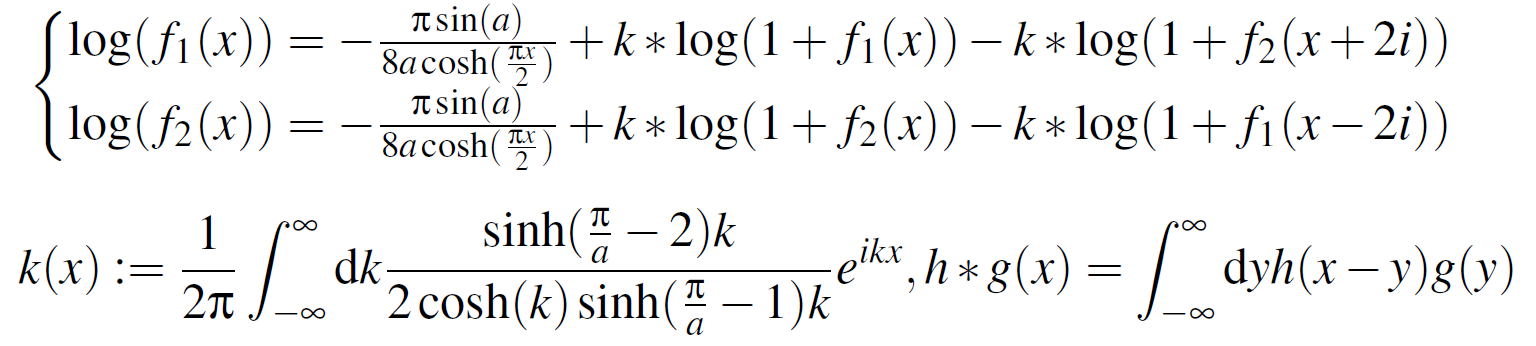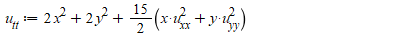## something wrong with a function in a procedure...

Hello!

I have a difficulty with a function used in procedure. The procedure uses a multivariable function and if the specific choice of the function is not made the procedure seems to give proper result, but In case I make a specific choice of the function and then try use this procedure gives me incorrect result.

To be more exact I use Physics package (there is a need to calcute combinations of covariant derivates ). The calculations are performed in a curved space with a defined metric.

So here is the procedure:

SD2 := proc (psi) SumOverRepeatedIndices(g_[~kappa, ~lambda]*(d_[kappa](d_[lambda](psi(X)))-Christoffel[~sigma, kappa, lambda]*d_[sigma](psi(X))))^2-SumOverRepeatedIndices(g_[~kappa, ~rho]*(d_[kappa](d_[lambda](psi(X)))-Christoffel[~sigma, kappa, lambda]*d_[sigma](psi(X)))*g_[~lambda, ~tau]*(d_[rho](d_[tau](psi(X)))-Christoffel[~gamma, rho, tau]*d_[gamma](psi(X)))) end proc;

If I turn to the procedure :

SD2(psi);

the result is  correct.

But  then I specify a psi function:

psi:=(t,r,x,y,z)->chi(r)+q*t;(here q is supposed to be a constant)

and turn to the procedure once again:

SD2(psi);

It gives me a wrong result.

I don't know what is the reason.

Thank you.

## How to solve Boundary value problem in set of eq...

System of equations with boundary conditions are moving?

Also, find the values of unknown variables?

## Can someone solve all of this questions into maple...## How do I solve numerically a set of non-linear int...

Hey everyone,

f_1 and f_2  are satisfying the set of non-linear integral equations I have attached to this message.
I know that I need to solve them numerically by iterations. Probably, the first guest of the function f_1 and f_2  is the driving term. a is just a parameter which can be fixed (I guess smaller than \pi/4). * is the convolution product and k is the momentum space parameter. I learnt that in order to solve them I should solve them in the Fourier space. I know also that I need to discretize these function in the “real ” space between {-L,+L} before applying the FFT or one of its relatives. Thank you for any suggestions or leads.## How to find triplets in Lie algebra at which Jacob...

Dear all

I have  Lie commutations for vectors e1, e2, e3, e4, e5, e6 as follow:

[e1, e3] = e3, [e1, e4] = e4, [e1, e5] = e5, [e1, e6] = e6, [e2, e3] = -e5, [e2, e4] = e6, [e3, e5] = e6

for which the command

Query("Jacobi")

returns the false result, which means, the vectors are not closed under Jacobi's identity. How can I find vector triplets for which Jacobi's identity does not hold?

## Error of Hfloat, Newtons iterations not converging...

 >>(1)
 >(2)
 >(3)
 >(4)
 >>## I encountered an error of Newtons iteration not co...

 >>(1)
 >(2)
 >(3)
 >(4)
 >>## How to find sgn on maple?...

How to find sgn on maple?

signum.mw

## Help me to solve the error of p2...

 >>>>>>>>>>(1)
 >(2)
 >>(3)
 >(4)
 >>>>>>>(5)
 >## what did i do wrong?...

 >>>>>## Maple Fails during a while cycle - Why?...

Hello, I found this very strange behavior in Maple and I can't explain why this is not working

A little bit of context first:

1. I placed a polygon as a boundary for a section and then two polygons that correspond to inner holes to the first one.
2. Then I proceed to create a regular mesh that places N x M points spread across the region (OK here)
3. A procedure checks weather or not a Point is inside the region, on the borders or in the inner holes (OK here)
4. Then I want to select the closest (but exterior) ones so I can create a path using the mesh points.

Here is where I find the issue. I start at a vertex called V[1,1] and I want to arrive at V[1,2] (in the document they are blue solid points marked they are near-vertices near the original polygons). The procedure that I apply finds a path towards the V[1,2] as I was expecting but when the procedure hits the V[1,2] coordinates the while loop does not stop

The condition I wrote was:    while point<>V[1,2] do  where 'point' is correctly generated by another procedure.

This happens in a 40 by 50 mesh but with other configurations it works properly (there are also another kind of errors but it's inherent to lack of optimization in my procedures).

What baffles me is why doesn't it stop the cycle, if I test the condition outside the loop it DOES recognize the points are indeed equal. Is it something in Maple or is it something in my PC?

Thanks to anyone who can see the error or the problem because I don't understand the nature of the problem.

Not_Working.mw

## Problem with pdsolve/numeric...

Problem with pdsolve/numeric

Contextualizing:

I created a routine for solving a thermo-mechanical problem. The size of the spatial domain is a function of time and the thermo-mechanical behavior of the structure.

The solution is obtained by discretizing the time domain in n intervals. The thermo-mechanical responses are obtained at each time t[i] =t[i-1]+dt.

The material exhibits elastic and elastoplastic mechanical behavior and thus the problem was divided into two consecutive phases (phase 1 and 2).

The heat problem is nonlinear because the thermal properties are variable (termal conductivity, specific heat, and density).

The problem:

In phase 2, the pdsolve/numeric command returns an error for the solution of the heat conduction equation (equation called pde1). I believe this error is related to the derivative of thermal conductivity k(x1) (piecewise function).

I tested many alternatives (I wrote the pde1 equation in two different ways), I checked the routine many times and I don't understand the reason for the error. With each attempt, the command returns a different error.

Thanks for your attention and help.

Problem.mw

## Differential Transform Method...

Could anyone help me out to convert the equation into differential transform method## How do I remove this error ''Error, (in dsolve/nu...

Please can you help me in resolving this error?

Here is the codeOptimal_control_model_of_DF_and_LP_2.mw